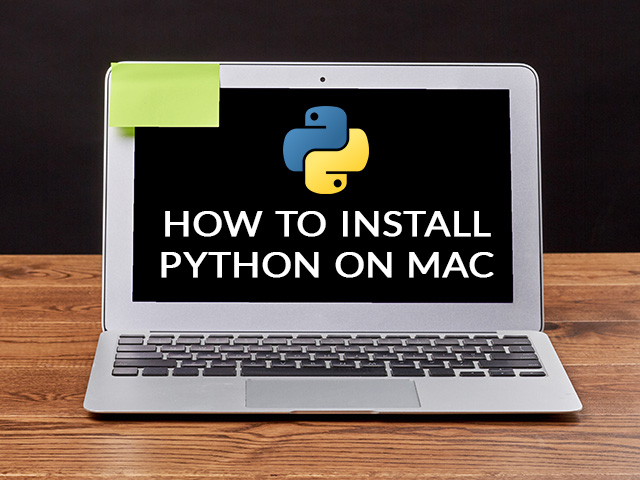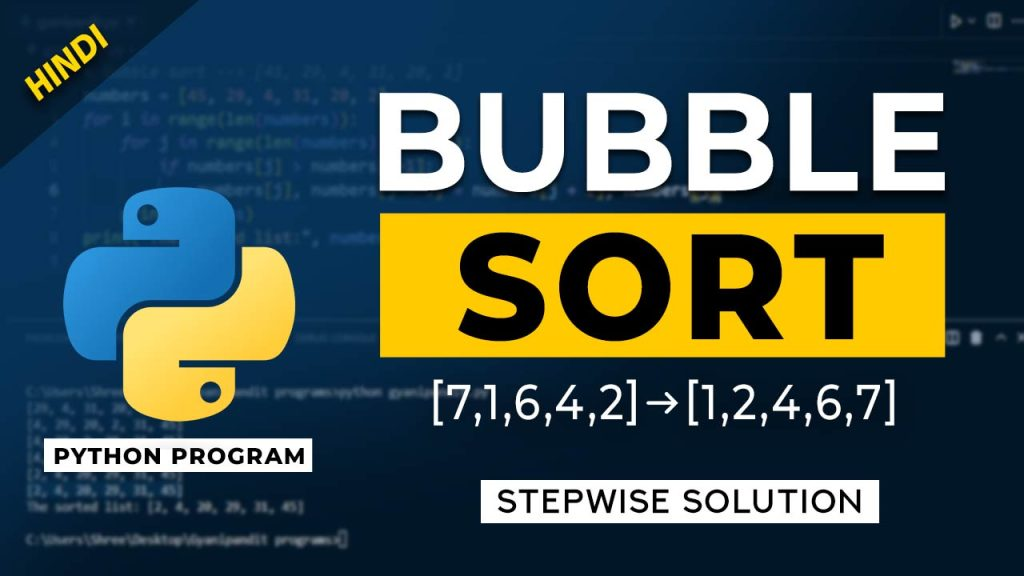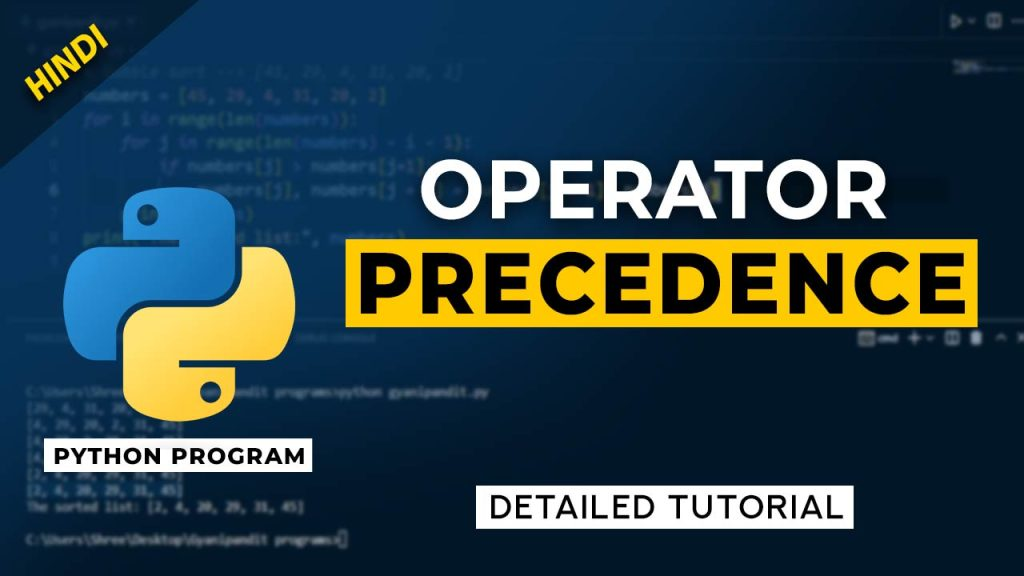# Python Tutorial## What is an str method in Python?

In this article, we are going to understand the __str__ method in Python. This method can be said as the dunder str method, where the dunder means double underscores. These methods with double underscores are also called magic methods. So, the __str__ method returns the string representation of the object. Basically, the __str__ method is …## How to install Python on mac

Python programming language is one of the most popular and powerful programming languages, and its uses are widespread in the areas of Data Science, Machine Learning, Application Development, Web Development, etc. So, if you are willing to learn Python programming language, you might want to get the latest version of python installed in your systems. …## Swap First and Last Digit of a Number in Python

In this video, we are going to understand and implement a simple Python program, where we are going to swap the first and last digits in the given number. For example, if we have the number 12345, the output should be 52341, where the first and last digits are swapped in the number. The logic …## Python program for bubble sort

In this video, we are going to understand a very simple sorting algorithm called Bubble sort, and we are going to implement a Python program, where we would use the bubble sorting technique, to sort our list. There are many sorting algorithms that you can explore. Here, in the bubble sorting algorithm, we are going …## Operator precedence in Python with Details

In this video, we are going to understand operator precedence in Python. This is very useful when we are dealing with multiple different operators in some expression. When we are using multiple operators in an expression, precedence decides what operation would be performed first in the expression. The precedence table can be found in the …